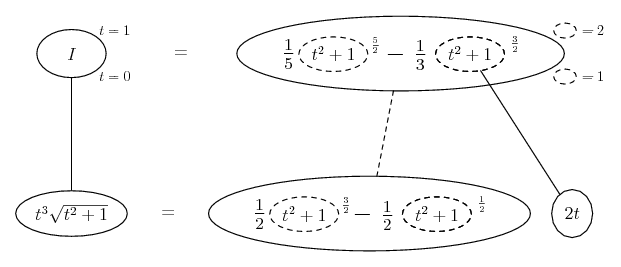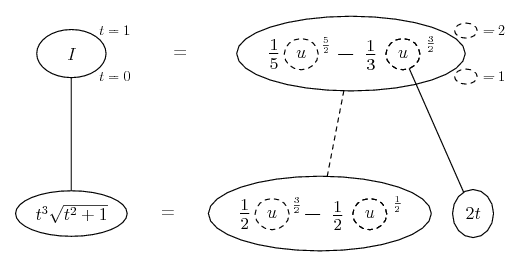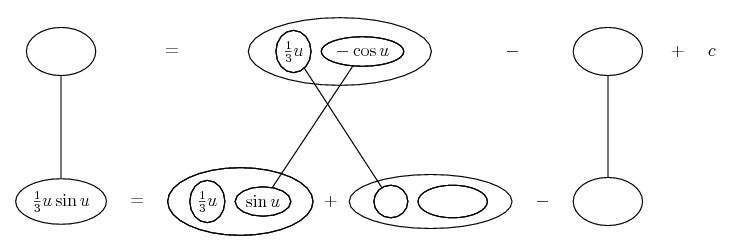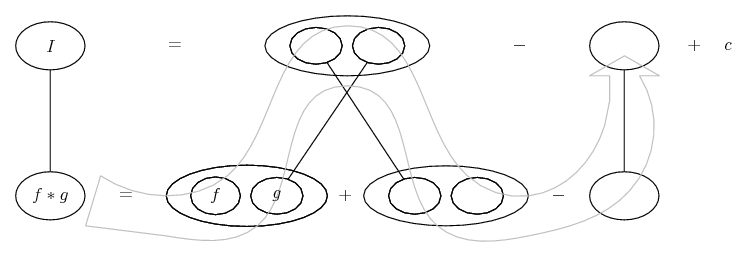1. ## Integration

I am having problem when it come to integration

(0 to 1) t^3 x sqrt(t^2 +1) dt

I try to use u substitution saying that

u= t^2 +1
du = 2t dt

then i don't know what to do because when I plug it in
it is like

(0 to 1) (1/2t) t^3 x sqrt(u) du
(0 to 1) (1/2) t^2 x sqrt(u) du

I dont' know what to do with t^2

-----------------------------------------------------------
And same with this problem
(0 to 1) t sin(3+3t) dt

so I try to use u substitution like
u= 3+3t
du= 3 dt

then I plug it in
(0 to 1) (1/3) t sin(u) du

and I got same problem again. I don't know what to do with t

------------------------------------------------------------
Am i using wrong way of integration? I am not really sure what am i doing wrong? I just can't continue

2. Both are fine (but watch your brackets). Next step is to use u-in-terms-of-t to express t in terms of u.

3. ##Originally Posted by tom@ballooncalculusBoth are fine (but watch your brackets). Next step is to use u-in-terms-of-t to express t in terms of u.
So You mean I should say....

u= t^2 +1
du = 2t dt

(0 to 1) (1/2t) t^3 x sqrt(u) du
(0 to 1) (1/2) t^2 x sqrt(u) du
(1/2) ∫(0 to 1) (u-1) x sqrt(u) du

(1/2) [ {(u^2/2 -u) x sqrt(u)} + {(u-1) x (2/3)(u^(3/2))|(0 to 1) ]

then I plug
u= (t^2 +1)

(1/2) [ {((t^2 +1)^2/2-(t^2 +1)) x sqrt((t^2 +1))} + {((t^2 +1)-1) x (2/3)((t^2 +1)^(3/2))|(0 to 1) ]

(1/2) ( [ {((1^2 +1)^2/2-(1^2 +1)) x sqrt((1^2 +1))} + {((1^2 +1)-1) x (2/3)((1^2 +1)^(3/2)) ] - [ {((0^2 +1)^2/2-(0^2 +1)) x sqrt((0^2 +1))} + {((0^2 +1)-1) x (2/3)((0^2 +1)^(3/2)) ]

(1/2) ( (2/3)(2^(3/2)) ] +(1/2) )

(1/3) (2^(3/2)) + (1/4)

is that right?

4.Originally Posted by uptown.cheekySo You mean I should say....

u= t^2 +1
du = 2t dt

(0 to 1) (1/2t) t^3 x sqrt(u) du
(0 to 1) (1/2) t^2 x sqrt(u) du
(1/2) ∫(0 to 1) (u-1) x sqrt(u) du

(1/2) [ {(u^2/2 -u) x sqrt(u)} + {(u-1) x (2/3)(u^(3/2))|(0 to 1) ]

You're thinking product rule, which if you were really thorough about it would become integration by parts... but far easier here is to multiply out the brackets in (1/2) ∫(0 to 1) (u-1) * sqrt(u) du and then apply the power rule, which you seem to know. Don't forget to re-set the limits for u, also. Indeed, then there's no need to convert back to t - you can evaluate the integral directly.

$\displaystyle \int_1^2 (u-1) u^{\frac{1}{2}}\ du = \int_1^2 (u^{\frac{3}{2}} - u^{\frac{1}{2}})\ du = ...$

Edit:

Just in case a picture helps...... or maybe... where... is the chain rule. Straight continuous lines differentiate downwards (integrate up) with respect to t, and the straight dashed line similarly but with respect to the dashed balloon expression (the inner function of the composite which is subject to the chain rule).

__________________________________________

Don't integrate - balloontegrate!

http://www.ballooncalculus.org/examples/gallery.html

http://www.ballooncalculus.org/asy/doc.html

5.Originally Posted by tom@ballooncalculusYou're thinking product rule, which if you were really thorough about it would give become integration by parts... but far easier here is to multiply out the brackets in (1/2) ∫(0 to 1) (u-1) * sqrt(u) du and then apply the power rule, which you seem to know. Don't forget to re-set the limits for u, also. Indeed, then there's no need to convert back to t - you can evaluate the integral directly.

$\displaystyle \int_1^2 (u-1) u^{\frac{1}{2}}\ du = \int_1^2 (u^{\frac{3}{2}} - u^{\frac{1}{2}})\ du = ...$
oh that make sense . Sound a lot easier now . Thanks
ok so for the 2nd one do i just use the product rule though?

6. Well, you do need to express t in terms of u again, and then expand the brackets just like in the first problem. And, again, the problem splits into the sum of two separate integration problems. However, one of these is a product that you DO need to tackle as such (there being no brackets to expand) but, as I said, to do this properly means doing 'integration by parts'.

Your two sub-problems are going to be (but see how you get here)

$\displaystyle \int \frac{1}{3} \sin u$ ... easy enough, and

$\displaystyle \int \frac{1}{3} u \sin u$ ... by parts. I'll post a pic.

Edit:

Just in case a picture helps...... where... is the product rule (straight continuous lines differentiate downwards (integrate up) with respect to u), and...... is integration by parts, which is a matter of satisfying the product rule, but starting in one of the bottom corners of the product rule shape instead of (as happens in differentiation) the top one. See if you can fill in the remaining blanks in the product-rule shape, then see if you can see the logic of having to subtract what you've just (in effect) added to the integrand. To keep the lower equals sign valid, in other words. Hope that helps. Yell if not!
__________________________________________

Don't integrate - balloontegrate!

http://www.ballooncalculus.org/examples/gallery.html

http://www.ballooncalculus.org/asy/doc.html

7. Super duper helpfulthanks

#### Search Tags

anti-derivative, calculas, integral, integration, u substitution